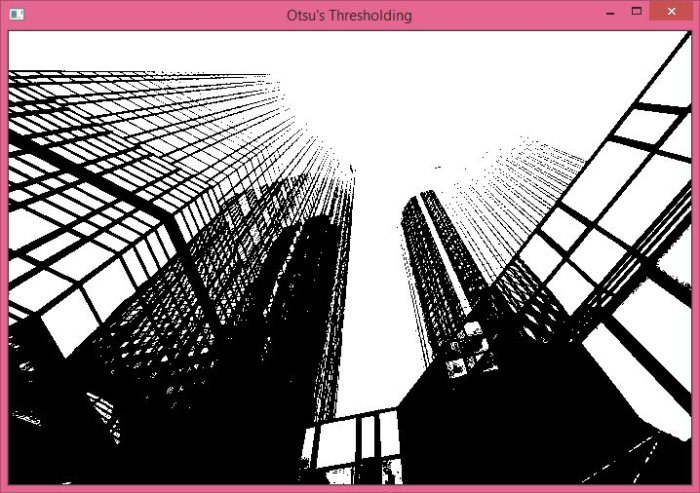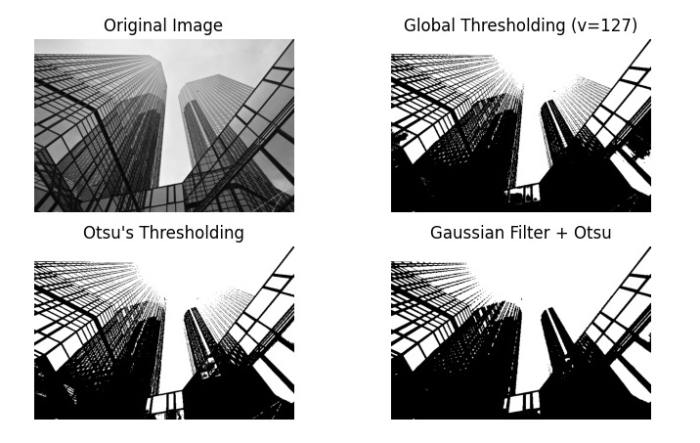# How to perform Otsu's thresholding on an image using Python OpenCV?

Otsu’s thresholding is a kind of thresholding technique. There are other types of thresholding techniques such as simple thresholding and adaptive thresholding.

The simple thresholding technique uses a global threshold value while the adaptive thresholding technique uses different threshold values for different regions.

Otsu’s thresholding technique uses a global threshold value but it is not chosen. It is determined automatically. It works accurately for bimodal images. The bimodal images are those images whose histogram has two peaks. The threshold value is the approximate value of the middle of these two peaks. If the image is not bimodal this thresholding is not accurate.

To apply Otsu’s thresholding, we apply simple thresholding cv2.threshold() with extra flag cv2.THRESH_OTSU. See the syntax given below.

## Syntax

cv2.threshold(img, thresh_val, max_valu, thresh_techniques)


## Parameters

• img − Input gray scale image. It's a numpy.ndarray.

• thresh_val − Threshold value. If the pixel value is higher than the threshold value, it is assigned one value else another value.

• max_valu − Maximum value to be assigned to a pixel

• thresh_techniques − Thresholding technique used. We can apply any simple thresholding + Otsu’s thresholding, i.e., cv2.THRESH_BINARY + cv2.THRESH_OTSU

It returns the globally adapted threshold value and the threshold.

Let's understand Otsu’s thresholding with the help of some Python examples

## Input Image

We will use this image as the input file in the following examples.## Example 1

In this program, we apply Otsu’s thresholding on the input image.

# import required libraries
import cv2

# read the input image as a gray image

# Apply Otsu's thresholding
_,th = cv2.threshold(img,0,255,cv2.THRESH_BINARY+cv2.THRESH_OTSU)

# display the output image
cv2.imshow("Otsu's Thresholding", th)
cv2.waitKey(0)
cv2.destroyAllWindows()


## Output

When you run the above program, it will produce the following output −The above output shows the image after applying Otsu’s Thresholding.

## Example 2

In this program, we apply Otsu’s thresholding on the input image. We also apply global thresholding and gaussian filter + Otsu’s thresholding.

import cv2

from matplotlib import pyplot as plt

# Apply global (simple) thresholding on image
ret1,th1 = cv2.threshold(img,127,255,cv2.THRESH_BINARY)

# Apply Otsu's thresholding on image
ret2,th2 = cv2.threshold(img,0,255,cv2.THRESH_BINARY+cv2.THRESH_OTSU)

# Apply Otsu's thresholding after Gaussian filtering
blur = cv2.GaussianBlur(img,(5,5),0)
ret3,th3 = cv2.threshold(blur,0,255,cv2.THRESH_BINARY+cv2.THRESH_OTSU)

titles = ['Original Image','Global Thresholding (v=127)',"Otsu's Thresholding",'Gaussian Filter + Otsu']
images = [img,th1,th2,th3]

for i in range(4):
plt.subplot(2,2,i+1)
plt.imshow(images[i], 'gray')
plt.title(titles[i])
plt.axis("off")
plt.show()


## Output

When you run the above program, it will produce the following output −The above output shows the different images after applying global thresholding, Otsu’s thresholding and Gaussian filters + Otsu’s thresholding.

Updated on: 27-Sep-2022

3K+ Views